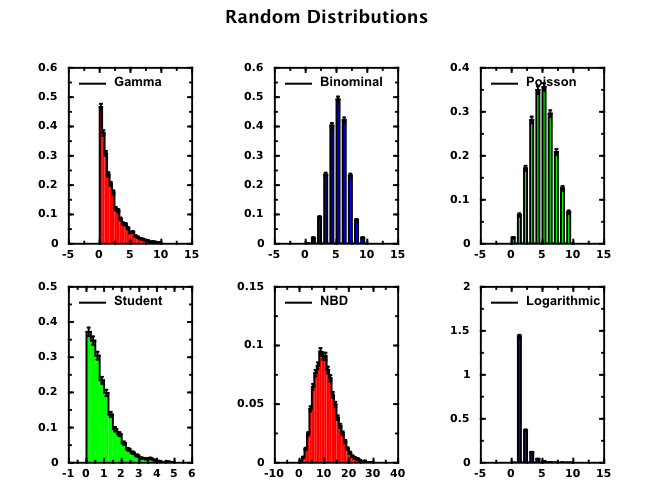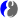# DScience:Discrete probability distributions and their characteristics

Limitted access. Login to DataMelt if you are a full DataMelt member.

30% complete

# What is discrete probability distribution?

Discrete probability distribution is a Probability_distribution of a discrete random variable X. Each possible value of the discrete random variable can be associated with a non-zero probability. A discrete probability distribution is often presented as tables.

Examples:

• The number of people going to a given shop per day.
• The number of students that come to class on a given day.

# Implementations

A number of Java libraries are available to create random discrete distributions:

Here is the code how to fill histograms using a number of popular distributions, including discrete:with the code;

= Description of discrete distributions

DataMelt can be used to determine statistical characteristics of an arbitrary frequency distribution, with moments calculated up to the 6th order. Read more aboutMoment (mathematics).

<pycode"> from jhplot import * from jhplot.math.StatisticSample import * a=randomLogNormal(1000,0,10) # generate random 1000 numbers between 0 and 10 using a LogNormal distribution p0=P0D(a) # convert it to an array print p0.getStatString() # print detailed characteristics </pycode>

To show this output, click expand

```Size: 1000
Sum: 2.0795326321690155E11
SumOfSquares: 1.722072831288292E22
Min: 4.3681673233597326E-14
Max: 1.187289072883721E11
Mean: 2.0795326321690154E8
RMS: 4.1497865382309628E9
Variance: 1.7194678431631995E19
Standard deviation: 4.14664664899627E9
Standard error: 1.3112848062732975E8
Geometric mean: 0.7193930848008395
Product: 9.252494313364321E-144
Harmonic mean: 2.2976022239249118E-11
Sum of inversions: 4.352363475222163E13
Skew: 25.65476598759878
Kurtosis: 694.7699433878664
Sum of powers(3): 1.839916709064571E33
Sum of powers(4): 2.0782654881247146E44
Sum of powers(5): 2.4093597349729484E55
Sum of powers(6): 2.8286717081193334E66
Moment(0,0): 1.0
Moment(1,0): 2.0795326321690154E8
Moment(2,0): 1.722072831288292E19
Moment(3,0): 1.839916709064571E30
Moment(4,0): 2.0782654881247147E41
Moment(5,0): 2.409359734972948E52
Moment(6,0): 2.8286717081193336E63
Moment(0,mean()): 1.0
Moment(1,mean()): 4.931390285491944E-7
Moment(2,mean()): 1.7177483753200437E19
Moment(3,mean()): 1.8291913748162454E30
Moment(4,mean()): 2.0630054468429083E41
Moment(5,mean()): 2.3878300421487077E52
Moment(6,mean()): 2.798744135044988E63
25%, 50%, 75% Quantiles: 0.0012310644573427145, 0.9530465118707188, 535.0653267374155
quantileInverse(median): 0.5005
Distinct elements & frequencies not printed (too many).
```

Let us continue with this example and now we would like to return all statistical characteristics of the sample as a dictionary. We can do this by appending the following lines that 1) create a dictionary "stat" with key/value pairs; 2) retrieve a variance of the sample using the key ``Variance.

which will print "Variance= 757.3". If not sure about the names of the keys, simply print the dictionary as "print stat".

One can create histograms that catch the most basic characteristics of data. This is especially important if there is no particular reasons to deal with complete data arrays. We can easily do this with above Fibonacci sequence as:

The code converts the array into a histogram with 10 equidistant bins in the range 0-100, and then it prints the map with statistical characteristics.

You can also visualize the random numbers in the form of a histogram as shown in this detailed example above. We create random numbers, convert them to histograms and plot them.

# Statistics with 2D arrays

You can get detailed statistics on data described byjhplot.P1D class using the method getStat(axis), where axis=0 for X and axis=1 for Y. It returns a map (for JAVA) or Python dictionary (for Jython) where each statistical characteristics can be accessed using a key, such as mean, RMS, variance, error on the mean at. Assuming that P1D is represented by "p1" object, try this code:

This will print the following values:

```error       0.996592835069
rms 	        5.05682000584
mean 	4.42857142857
variance 	6.95238095238
stddev 	 2.63673679998```

Here is a more detailed example:

This tutorial is provided under this license agreement.